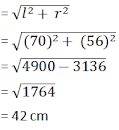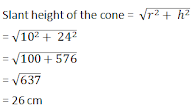# ICSE Solutions for Chapter 20 Cylinder Cone and Sphere Class 10 Mathematics

Question 1: Find the volume of a cone whose slant height is 17 cm and radius of base is 8 cm.

Solution 1: Slant height (l) = 17 cm
But,
l2 = r2 + h2
⇒ h2 =  l2 - r2
⇒ h2 = 172 – 82
⇒ h2 = 289 – 64 = 225 - (15)2
∴ h = 15
Now, volume of cone = 1/3πr2h
= 1/3 × 22/7 × 8 × 8 × 15 cm3
= 7040/7 cm3
= 1005.71 cm3

Question 2: The curved surface area of a cone is 12320 cm2. If the radius of its base is 56 cm, find its height.

Solution 2: Curved surface area = 12320 cm2
Radius of base (r) = 56 cm
Let slant height = l
∴ πrl  = 12320
⇒ 22/7 × 56 x l = 12320
⇒ l = (12320 x 7)/(56 × 22)
⇒ l = 70 cm
Height of the cone =Question 3: The circumference of the base of a 12 m high conical tent is 66 m. Find the volume of the air contained in it.

Solution 3: Circumference of the conical tent = 66 m
and height (h) = 12 m
∴ Radius = c/2π = (66 × 7)/(2 × 22) = 10.5 m
Therefore, volume of air contained in it = 1/3πr2h
= 1/3 × 22/7 × 21/2 × 21/2 × 12m3
= 1386 m3

Question 4: The radius and height of a right circular cone are in the ratio 5:12 and its volume is 2512 cubic cm. Find the radius and slant height of the cone. (Take π = 3.14)

Solution 4: The ratio between radius and height = 5:12
Volume = 2512 cubic cm
Let radius (r) = 5x, height (h) = 12x and slant height = l∴ l2 = r2 + h2
⇒ l2 = (5x)2 + (12x)2
⇒ l2 = 25x2 + 144x2
⇒ l2 = 169x2
⇒ l2 = 13x
Now Volume = 1/3πr2h
⇒ 1/3πr2h = 2512
⇒ 1/(3.14)(5x)2(12x) = 2512
⇒ 1/3(3.14)(300x3)
∴ x3 = (2512 × 3)/(3.14 × 300) = (2512×3×100)/(314×300) = 8
⇒ x = 2
∴ Radius = 5x = 5 × 2 = 10
Height = 12x =  12 × 2 = 24 cm
Slant height = 13x = 13 × 2 = 26 cm

Question 5: 8 metallic sphere; each of radius 2 mm, are melted and cast into a single sphere. Calculate the radius of the new sphere

Solution 5: Radius of metallic sphere = 2 mm = 1/5 cm
Volume = 4/3πr3 = 4/3 × 22/7 × 1/5 × 1/5 × 1/5 = 88/(21 × 125) cm3
Volume of 8 spheres = (88 × 8)/(21 × 125) = 704/(21×125)cm3 ...(i)
Let radius of new sphere = R
∴ Volume = 4/3πR3 = 4/3 × 22/7 R3 = 88/2R...(ii)
From (i) and (ii)
88/21R3 = 704/(21 × 125)
⇒ R3 = 704(21 × 125) × 21/88 = 8/125
⇒ R = 2/5 = 0.4 cm = 4 mm

Question 6: The volume of one sphere is 27 times that of another sphere. Calculate the ratio of their
(ii) surface areas

Solution 6:
Volume of first sphere = 27 × volume of second sphere
Let radius of first sphere = r1
and radius of second sphere = r2
Therefore, volume of first sphere = 4/3 πr13
and volume of second sphere = 4/3 πr23

(i) Now, according to the question
4/3πr13 = 27 × 4/3πr23
r13 = 27r23 = (3r2)3
⇒ r1 = 3r2
⇒ r1/r2 = 3/1
∴ r1 : r2 = 3 : 1

(ii) Surface area of first sphere = 4πr12
and surface area of second sphere = 4πr22
Ratio in surface area = 4πr12/4πr22 = r12/r22 = 32/12 = 9/1 = 9:1

Question 7: A wooden toy is in the shape of a cone mounted on a cylinder as shown alongside.If the height of the cone is 24 cm, the total height of the toy is 60 cm and the radius of the base of the cone is twice the radius of the base of the cylinder = 10 cm; find the total surface area of the toy. [Take π =  3.14]

Solution7:
Height of the cone = 24cm
Height of the cylinder = 36 cm
Radius of the cone-twice the radius of the cylinder = 10 cm
Radius of the cylinder = 5 cmNow, the surface area of the toy = curved area of the conical point + curved area of the cylinder
= πrl + πr2 + 2πRH
= π[rl + r2 + 2RH]
= 3.14[10 × 26 + (10)2 + 2×5×36]
= 3.14[260 + 100 + 360]
= 3.14×
= 2260.8 cm2

Question 8: In the following diagram a rectangular platform with a semicircular end on one side is 22 meters long from one end to the other end. If the length of the half circumference is 11 meters, find the cost of constructing the platform, 1.5 meters high at the rate of Rs 4 per cubic meters.Solution 8: Length of the platform = 22m
Circumference of semicircle  = 11m
∴ Radius = (c × 2)/(2 × π) = (11 × 7)/22 = 7/2m
Therefore, breadth of the rectangular part = 7/2 × 2 = 7m
And length = 22 – 7/2  = 37/2 = 18.5 m
Now area of platform = l × b + ½ πr2
= 18.5 × 7 + ½ × 22/7 × 7/2 × 7/2 m2
= 129.5 + 77/4 m2
= 148.75 m2
Height of the platform = 1.5 m
∴ Volume = 148.75 × 1.5 = 223.125 m3
Rate of construction = Rs 4 per m3
Total expenditure  = Rs 4 × 223.125 = Rs 892.50

Question 9: The total area of a solid metallic sphere is 1256 cm2. It is melted and recast into solid right circular cones of radius 2.5 cm and height 8 cm. Calculate:
(i) the radius of the solid sphere
(ii) the number of cones recasted [π = 3.14]

Solution 9: Total area of solid metallic sphere = 1256 cm2
(i) Let radius of the sphere is then
4πr2 = 1256
4 x 22/7r2 = 1256
⇒ r2 = (1256 ×7)/(4 × 22)
⇒ r2 = (157 × 7)/11
⇒ r2 = 1099/11
⇒ r = √99.909 = 9.995 cm
⇒ r = 10 cm

(ii) Volume of sphere = 4/3πr3 = 4/3 × 22/7 × 10 × 10 × 10 = 88000/21 cm3
Volume of right circular cone = 1/3πr2h = 1/3 × 22/7 × (2.5)2 × 8 = 1100/21 cm3
Number of cones = 88000/21 + 1100/21
= 88000/21 × 21/1100
= 80

Question 10: A solid metallic cone, with radius 6 cm and height 10 cm, is made of some heavy metal A. In order to reduce weight, a conical hole is made in the cone as shown and it is completely filled with a lighter metal B. The conical hole has a diameter of 6 cm and depth 4 cm. Calculate the ratio of the volume of the metal A to the volume of metal B in the solid.Solution 10: Volume of the whole cone of metal A = 1/3πr2h
= 1/3 × π × 62  × 10
= 120 π

Volume of the cone with metal B = 1/3πr2h
= 1/3 × π × 3× 4
= 12π
Final Volume of cone with metal A = 120π - 12π = 108π
Volume of cone with metal A/Volume of cone with metal B
=  108π/12π = 9/1